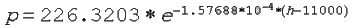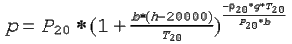Home
Balloons
Theory
Std. Atmosph.

Language:
EspaûÝol

More theory:
Standard atm.
Calculator
Descent spd

## Standard atmosphere

After searching quite a bit for practical formulae to do this job, I finally took the excellent work of Hugo Lorente, published by AMSAT Argentina in the proceedings of the 1er Simposio de Comunicaciones Satelitales y Digitales (22/23 de setiembre de 1990), and derived the ones that are below.
(Each case presents the theoretical formula, and then a version with all constants replaced by their values).

For those who are not interested in the formulas, I've published a an on-line calculator on another page.

OJO: All formulas use metric units! (like hectoPascal (hPa), g in m/sý, height in meters (m), etc.

 Presure > 226.3203 hPa54.749 < Pressure < 226.3203 hPa8.68 < Pressure < 54.749 hPaPressure < 8.68 hPaTo complete the list, I'll include the original (inverse) formulas below:

 Height < 11000m11000m < Height < 20000m20000m < Height < 32000m32000m < HeightWhere:

T0
Temperature at 0 m (288.15¯K)
T11
Temperature at 11000 m
T20
Temperature at 20000 m
T32
Temperature at 32000 m
a
Temperature at sea level
b
Temperature decrease per m altitude:
```         0 - 11000m   -0.0065¯K/m
11000 - 20000m   Stable
20000 - 32000m   +0.001¯K/m
32000 - 47000m   +0.0028¯K/m
```
g
9.80665 m/sý
rho0
Air density at 0 m (1.225 kg/m3)
P0
Pressure at 0 m (1013.25 hPa)

 (c) John Coppens ON6JC/LW3HAZ mail